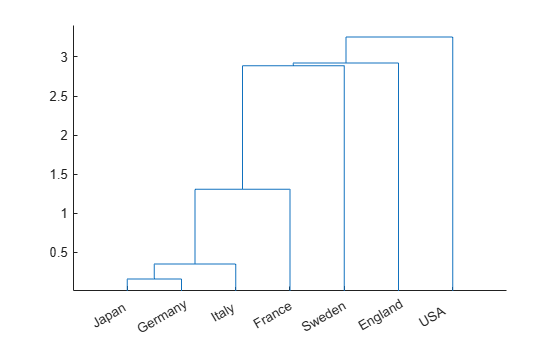# manovacluster

Dendrogram of group mean clusters following MANOVA

## Syntax

```manovacluster(stats) manovacluster(stats,method) H = manovacluster(stats,method) ```

## Description

`manovacluster(stats)` generates a dendrogram plot of the group means after a multivariate analysis of variance (MANOVA). `stats` is the output `stats` structure from `manova1`. The clusters are computed by applying the single linkage method to the matrix of Mahalanobis distances between group means.

See `dendrogram` for more information on the graphical output from this function. The dendrogram is most useful when the number of groups is large.

`manovacluster(stats,method)` uses the specified method in place of single linkage. `method` can be one of these values, which identify the methods used to create the cluster hierarchy. (See `linkage` for additional information.)

MethodDescription

`'single'`

Shortest distance (default)

`'complete'`

Largest distance

`'average'`

Average distance

`'centroid'`

Centroid distance

`'ward'`

Incremental sum of squares

`H = manovacluster(stats,method)` returns a vector of handles to the lines in the figure.

## Examples

collapse all

`load carbig`

Define the variable matrix.

`X = [MPG Acceleration Weight Displacement];`

Perform one-way MANOVA to compare the means of MPG, Acceleration, Weight,and Displacement grouped by Origin.

`[d,p,stats] = manova1(X,Origin);`

Create a dendrogram plot of the group means.

`manovacluster(stats)`## Version History

Introduced before R2006a